# Apollony Fractal

Written by Paul Bourke
June 2003

Computers and Graphics, Vol 30, Issue 1, January 2006, pages 134-136

The Apollony fractal is created by starting with three mutually tangential circles as shown below.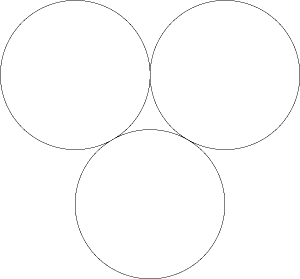There are two circles which have these three circles as their tangent, they are called the inner and outer Soddy circles. The derivation of the radius of the inner and outer Soddy circles were studied by Frederick Soddy around 1936. The Apollony fractal is created by repeatedly filling the gaps between the circles with the inner Soddy circle. The first two iterations are shown below.The following is the Apollony fractal iterated to a "depth" of 1000 circles. The normal interpretation is to consider the plane to be solid and the circles are cutting out holes, as such the area tends to zero.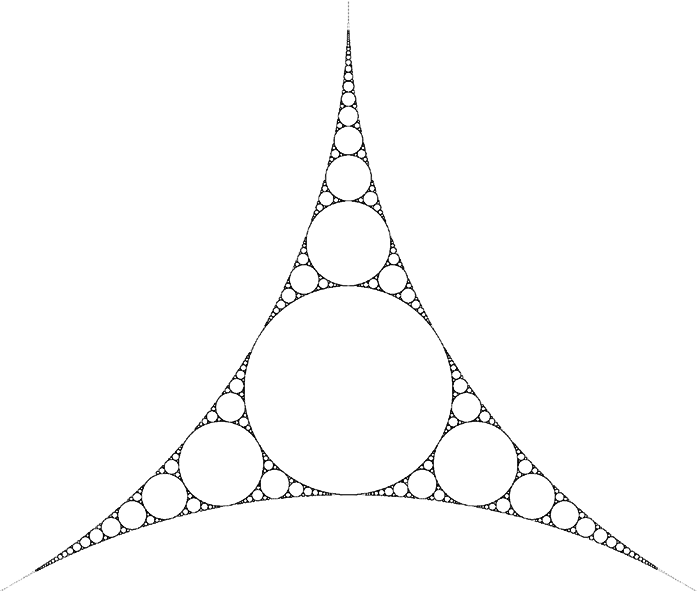The fractal dimension is approximately 1.3058. The name is attributed to Apollonius of Perga who first proved that given any 3 circles that there were 8 circles tangent to all three.

The Apollony fractal can be represented in 3D space by turning each circle into a sphere, three views of this are shown below (click on the small image for a larger version). Note that the three large spheres have been discarded so the internal structure is clearer.The Apollonian gasket is usually the name given to the form that results from the same process described above but with an outer Soddy circle around the three circles above. The inner Soddy circles are added in the same way noting that the outer circle can now be tangent to the added circles.The following is the gasket above with the circles drawn as spheres and the outer sphere and three initial spheres removed.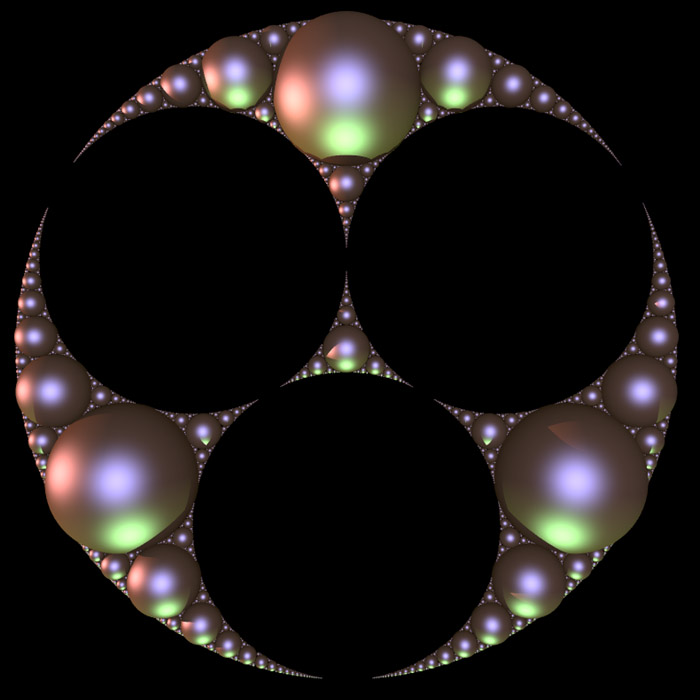3D

In 3D one starts with 4 spheres each at the vertices of a tetrahedron. A outer Soddy sphere is added that encloses the 4 spheres. The gaps are filled by subsequent Soddy spheres thus forming a solid object (at infinity). The result is often called an Apollonian sphere packing. The fractal dimension of the 3D Apollonian has been calculated as 2.473946 [M. Borkovec, W. De Paris, and R. Peikert].

The following shows the top half of the 3D Apollonian, with the outer and 4 tetrahedral spheres removed. As with most fractals, this is an approximation with "only" 40,000 spheres rather than the infinite number required for the true fractal.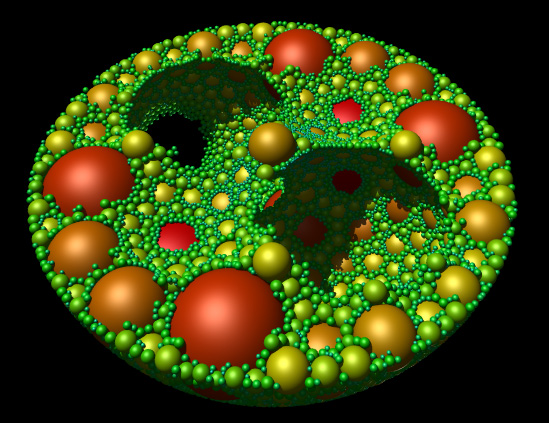This is the exterior of the whole Apollonian. Note the spheres are shaded with a red to blue colour ramp depending on their radius except for the 4 tetrahedral spheres that are grey.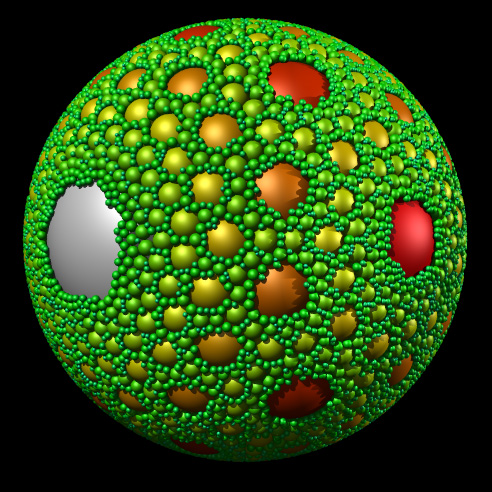And finally, a view from the inside with the 4 tetrahedral spheres removed of course. The first image is a standard perspective and the second is a 180 degree fisheye.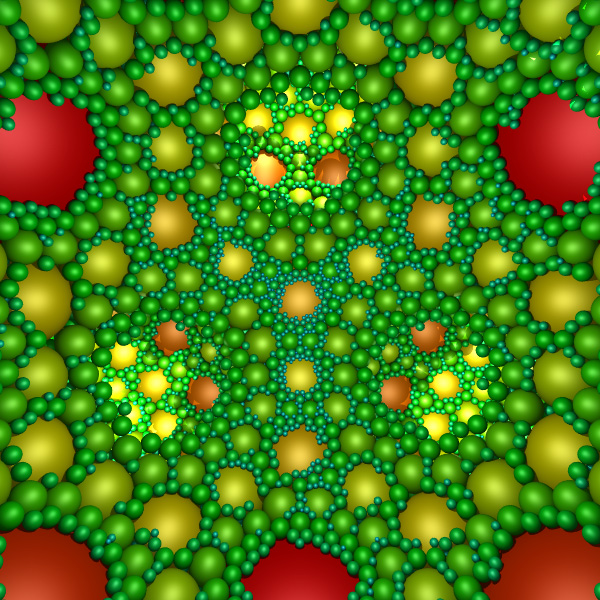Attractor

Kravchenko Alexei and Mekhontsev Dmitriy have found an attractor that creates the Apollony fractal, the source code in Basic (by Roger Bagula) and C (by myself) are provided here: roger.bas and apollony.c.

This is the union of three functions
 f1(z) = f(z) f2(z) = 0.5 (-1 + s i) / f(z) f3(z) = 0.5 (-1 - s i) / f(z)

where z is complex, s=sqrt(3), and

f(z) = 3 / ( 1 + s - z) - (1 + s) / (2 + s)

For those who need a refresher on complex division see this.Rendered as a landscape, flooded